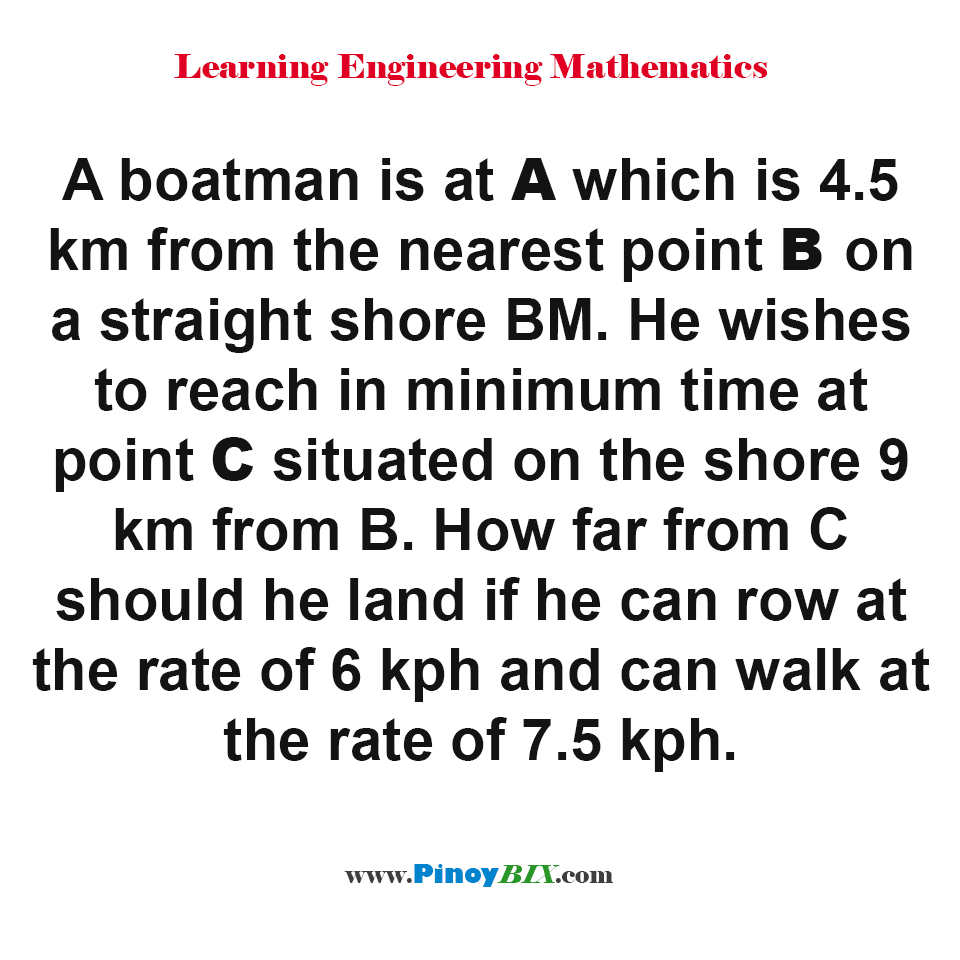# Solution: How far from C should he land if he can row at the rate of 6 kph and can walk at the rate of 7.5 kph

(Last Updated On: January 21, 2020)#### Problem Statement: EE Board October 1993

A boatman is at A which is 4.5 km from the nearest point B on a straight shore BM. He wishes to reach in minimum time at point C situated on the shore 9 km from B. How far from C should he land if he can row at the rate of 6 kph and can walk at the rate of 7.5 kph.

• A. 4.15 km
• B. 3.0 km
• C. 3.25 km
• D. 4.0 km

The distance from B to C is 3.0 km

#### Latest Problem Solving in Differential Calculus (MAXIMA-MINIMA & TIME RATES)

More Questions in: Differential Calculus (MAXIMA-MINIMA & TIME RATES)